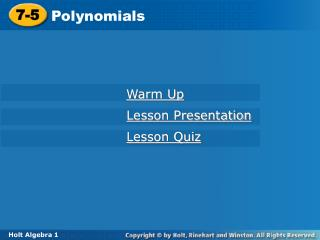DownloadDownload Presentation7-5

# 7-5

Télécharger la présentation## 7-5

- - - - - - - - - - - - - - - - - - - - - - - - - - - E N D - - - - - - - - - - - - - - - - - - - - - - - - - - -
##### Presentation Transcript

1. 7-5 Polynomials Warm Up Lesson Presentation Lesson Quiz Holt Algebra 1

2. Objectives Classify polynomials and write polynomials in standard form. Evaluate polynomial expressions.

3. A monomial is a number, a variable, or a product of numbers and variables with whole-number exponents. The degree of a monomial is the sum of the exponents of the variables. A constant has degree 0.

4. A. 4p4q3 Example 1: Finding the Degree of a Monomial Find the degree of each monomial. B. 7ed C. 3

5. a. b. c. 1.5k2m 4x 2c3 Check It Out! Example 1 Find the degree of each monomial.

6. A polynomialis a monomial or a sum or difference of monomials. The degree of a polynomial is the degree of the term with the greatest degree.

7. B. Example 2: Finding the Degree of a Polynomial Find the degree of each polynomial. A. 11x7 + 3x3

8. Check It Out! Example 2 Find the degree of each polynomial. a. 5x – 6 b. x3y2 + x2y3 – x4 + 2

9. The terms of a polynomial may be written in any order. However, polynomials that contain only one variable are usually written in standard form. The standard form of a polynomial that contains one variable is written with the terms in order from greatest degree to least degree. When written in standard form, the coefficient of the first term is called the leading coefficient.

10. Example 3A: Writing Polynomials in Standard Form Write the polynomial in standard form. Then give the leading coefficient. 6x – 7x5 + 4x2 + 9

11. Example 3B: Writing Polynomials in Standard Form Write the polynomial in standard form. Then give the leading coefficient. y2 + y6 − 3y

12. Remember! A variable written without a coefficient has a coefficient of 1. y5 = 1y5

13. Check It Out! Example 3a Write the polynomial in standard form. Then give the leading coefficient. 16 – 4x2 + x5 + 9x3

14. Terms Name 1 Monomial 0 Constant 2 Binomial 1 Linear 3 Trinomial Quadratic 2 Polynomial 4 or more Cubic 3 Quartic 4 Quintic 5 6 or more 6th,7th,degree and so on Some polynomials have special names based on their degree and the number of terms they have.

15. Example 4: Classifying Polynomials Classify each polynomial according to its degree and number of terms. A. 5n3 + 4n B. 4y6 – 5y3 + 2y – 9 C. –2x

16. Example 5: Application A tourist accidentally drops her lip balm off the Golden Gate Bridge. The bridge is 220 feet from the water of the bay. The height of the lip balm is given by the polynomial –16t2 + 220, where t is time in seconds. How far above the water will the lip balm be after 3 seconds?

17. Example 5: Application Continued A tourist accidentally drops her lip balm off the Golden Gate Bridge. The bridge is 220 feet from the water of the bay. The height of the lip balm is given by the polynomial –16t2 + 220, where t is time in seconds. How far above the water will the lip balm be after 3 seconds?

18. Check It Out! Example 5 What if…? Another firework with a 5-second fuse is launched from the same platform at a speed of 400 feet per second. Its height is given by –16t2 +400t + 6. How high will this firework be when it explodes?

19. Lesson Quiz: Part I Find the degree of each polynomial. 1. 7a3b2 – 2a4 + 4b –15 2. 25x2 – 3x4 Write each polynomial in standard form. Then give the leading coefficient. 3. 24g3 + 10 + 7g5 – g2 4. 14 – x4 + 3x2

20. Lesson Quiz: Part II Classify each polynomial according to its degree and number of terms. 5. 18x2 – 12x + 5 6. 2x4 – 1 7. The polynomial 3.675v + 0.096v2 is used to estimate the stopping distance in feet for a car whose speed is v miles per hour on flat dry pavement. What is the stopping distance for a car traveling at 70 miles per hour?